Next: Annexes Up: Conclusions Previous: Some advice on how   Contents

# The H-norm

The shape of an ideal trust region should reflect the geometry of the model and not give undeserved weight to certain directions.
Perhaps the ideal trust region would be in the H-norm, for which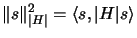(12.3)

and where the absolute value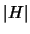is defined by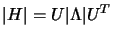, where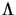is a diagonal matrix constituted by the eigenvalues ofand where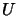is an orthonormal matrix of the associated eigenvectors and where the absolute value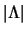of the diagonal matrixis simply the matrix formed by taking absolute values of its entries.
This norm reflects the proper scaling of the underlying problem - directions for which the model is changing fastest, and thus directions for which the model may differ most from the true function are restricted more than those for which the curvature is small.
The eigenvalue decomposition is extremely expensive to compute. A solution, is to consider the less expensive symmetric, indefinite factorization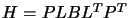(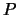is a permutation matrix,is unit lower triangular,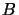is block diagonal with blocks of size at most 2). We will use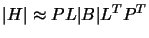with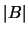computed by taking the absolute values of the 1 by 1 pivots and by forming an independent spectral decomposition of each of the 2 by 2 pivots and reversing the signs of any resulting negative eigenvalues.